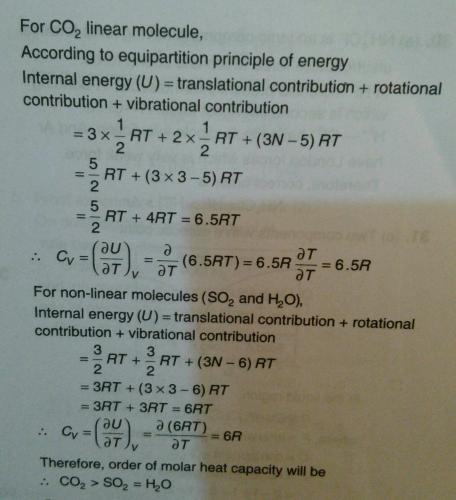Courses

# According to the equipartition principle of energy, the molar heat capacity at constant volume for CO2(g), SO2(g) and H2O(g) follows the trends:a)CO2 = SO2 = H2Ob)CO2 < SO2 = H2Oc)CO2 > SO2 = H2Od)CO2 = SO2 > H2OCorrect answer is option 'C'. Can you explain this answer? Related Test: Test: Gaseous State- 2

## Chemistry Question

By Sushmita · Jan 29, 2019 ·Chemistry
Vipin Singh answered Jan 29, 2019Yaseen Khan answered Oct 21, 2018
C is correct

Amit Rai answered Dec 28, 2018